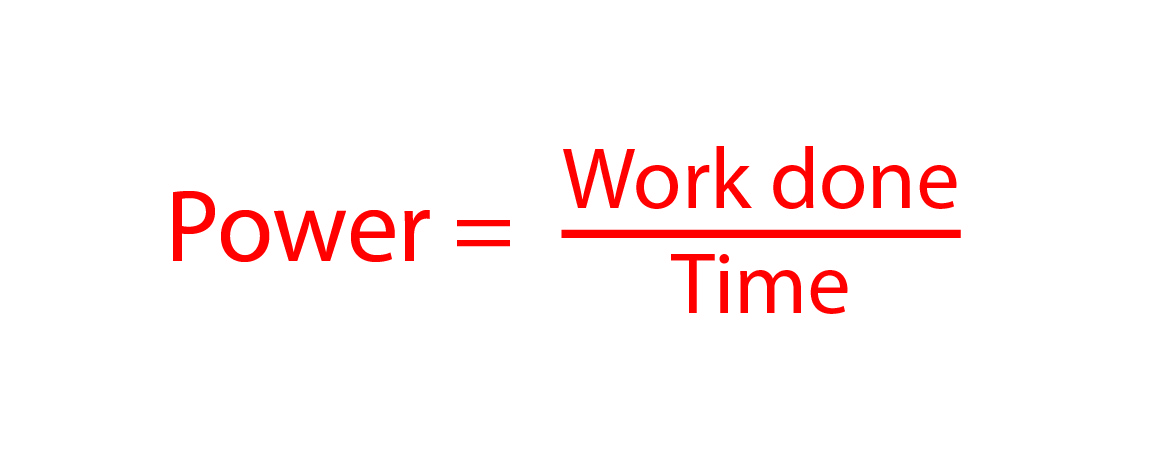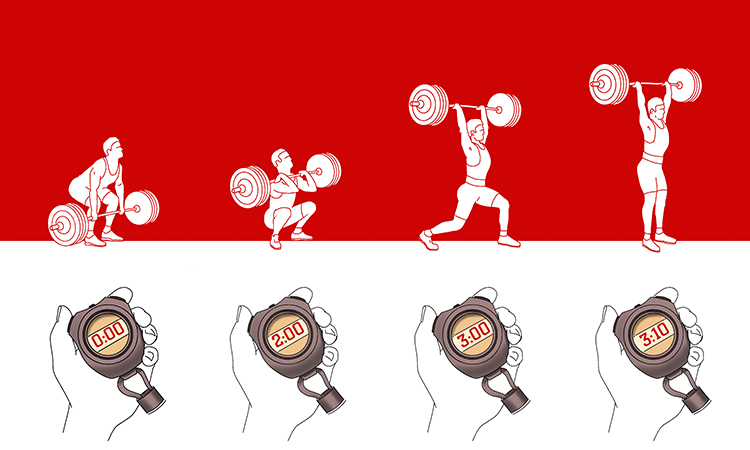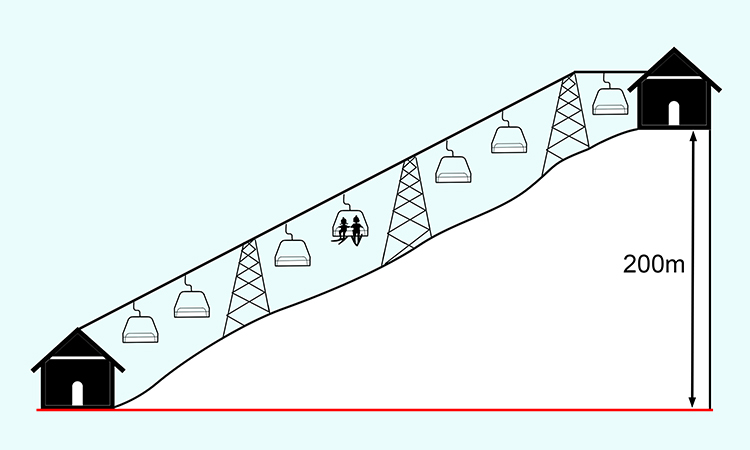# Power

Power is the measure of how much work is done (the distance an object is moved multiplied by the force required to do so) in a given time. The formula for power is:The Power lifter worked the weights to the top but it took over 3 minutes.

Alternatively

The Power lifter worked out in the gym over time to build his physique.

NOTE:

Power is measured in watts, or Joules per second.

Example 1

If a chair lift carries two skiers, Lindsey and Julia, 200m vertically up a mountain, with Lindsey weighing 700N and Julia 500N, what is the work done on them by the lift and if the journey takes 5 minutes what is the power required?Work done = energy transferred = gain in gravitational potential energy

W\o\r\k\ d\o\n\e = massxxgravityxxchan\g\e\ i\n\ height

Weight = massxxgravity                therefore:

W\o\r\k\ d\o\n\e = weightxxchan\g\e\ i\n\ height=(700+500)xx200=1200xx200=240,000\ Jo\u\l\e\s

Power=(w\o\r\k\ d\o\n\e)/(time)

Don’t forget to convert the time the lift takes into the standard units required for the formula. This means we must convert 5 minutes to seconds.

5xx60=300\ s\e\c\o\n\d\s

Power=(240,000)/300=800\ W\a\t\t\s

Example 2

If Lindsey uses 800 watts to ski back to the bottom of the lift and Julia 600 watts, how long does it take each skier to make it back to the base of the lift?

Power=(w\o\r\k\ d\o\n\e)/(time)

Multiply both sides by time to make work done the subject,

Powerxxtime=(w\o\r\k\ d\o\n\exxcancel(time))/(cancel(time))

Divide both sides by power to make time the subject,

(cancel(power)xxtime)/cancel(power)=(w\o\r\k\ d\o\n\e)/(power)

L\i\n\d\s\ey\ time=(w\o\r\k\ d\o\n\e)/(L\i\n\d\s\ey\ power)=(700xx200)/800=(140,000)/800=175\ se\c\o\n\ds

175\ se\c\o\n\ds = 2\ m\i\n\u\t\es\ 55\ s\e\c\o\n\ds

J\u\l\i\a\ time=(w\o\r\k\ d\o\n\e)/(J\u\l\i\a\ power)=(500xx200)/600=(100,000)/600=166.67\ se\c\o\n\ds

166.67\ s\e\c\o\n\ds = 2\ m\i\n\u\t\es\ 46.67\ s\e\c\o\n\ds